# Date game

Let now is Wednesday. What day is after 248 days?
Write the result as a number: 1=Monday, 2=Tuesday, 3=Wednesday, 4=Thursday, 5=Friday, 6=Saturday, 7=Sunday?

Result

d =  6

#### Solution:Leave us a comment of example and its solution (i.e. if it is still somewhat unclear...):Be the first to comment!## Next similar examples:

1. Cake 71/3 of a cake shared with 4 people. What share of the whole cake has each people?
2. ChocolateChildren break chocolate first to third and then every part of another half. What kind got each child? Draw a picture. What part would have received if each piece have halved?
3. Odometer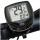The odometer is driven by rotation of the wheel whose diameter is 65 cm. After how many rotations the wheel turns counter to next kilometer?
4. Unit rateFind unit rate: 6,840 customers in 45 days
5. Four families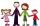Four families were on a joint trip. In the first family, there were three siblings, namely Alica, Betka and Cyril. In the second family were four siblings, namely David, Erik, Filip and Gabika. In the third family, there were two siblings, namely Hugo and
6. Fraction and a decimalWrite as a fraction and a decimal. One and two plus three and five hundredths
7. The resultHow many times I decrease the number 1632 to get the result 24?
8. Math classificationIn 3A class are 27 students. One-third got a B in math and the rest got A. How many students received a B in math?
9. Sweets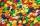Mom bought box of sweets for their children.Whole package of 100 sweets divided among 4 their children so that each child receives the most and for she remains the least sweets. How many sweets left for mom.
10. Pizza 5You have 2/4 of a pizza and you want to share it equally between 2 people how much pizza does each person get?
11. Fractions 4How many 2/3s are in 6?
12. Product of two fractionsProduct of two fractions is 9 3/5 . If one of the fraction is 9 3/7. Find the other fraction.
13. Crates 2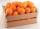One crate will hold 50 oranges. If Bob needs to ship 932 oranges, how many crates will he need?
14. Trio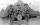56 children lined up in groups of three. How many children did not create a trio?
15. Lengths of the poolMiguel swam 6 lengths of the pool. Mat swam 3 times as far as Miguel. Lionel swam 1/3 as far as Miguel. How many lengths did mat swim?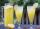How many 1/4 cup servings are in 2 and 1/3 cups of lemonade?Solve for a specified variable: P=a+4b+3c, for a